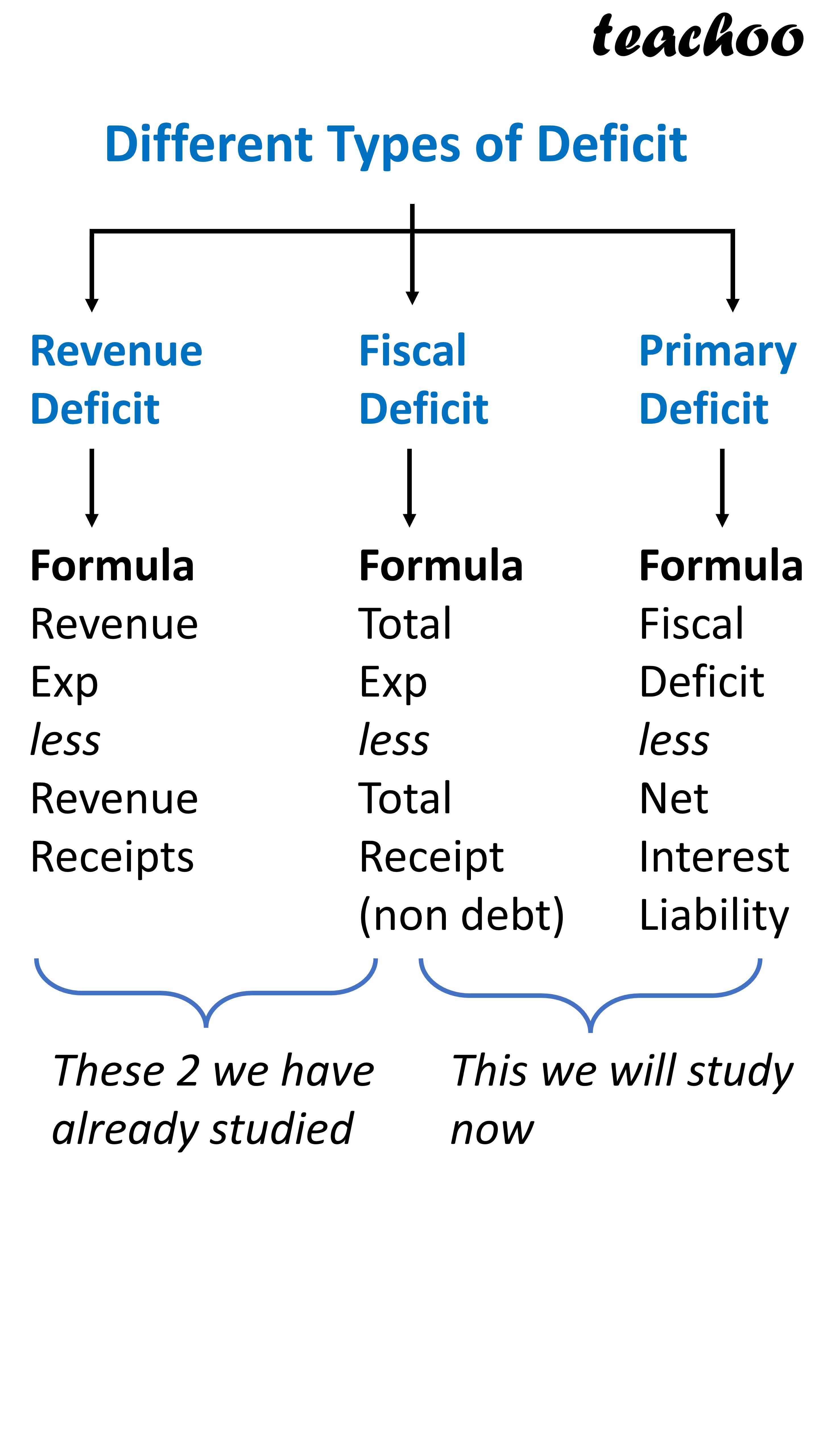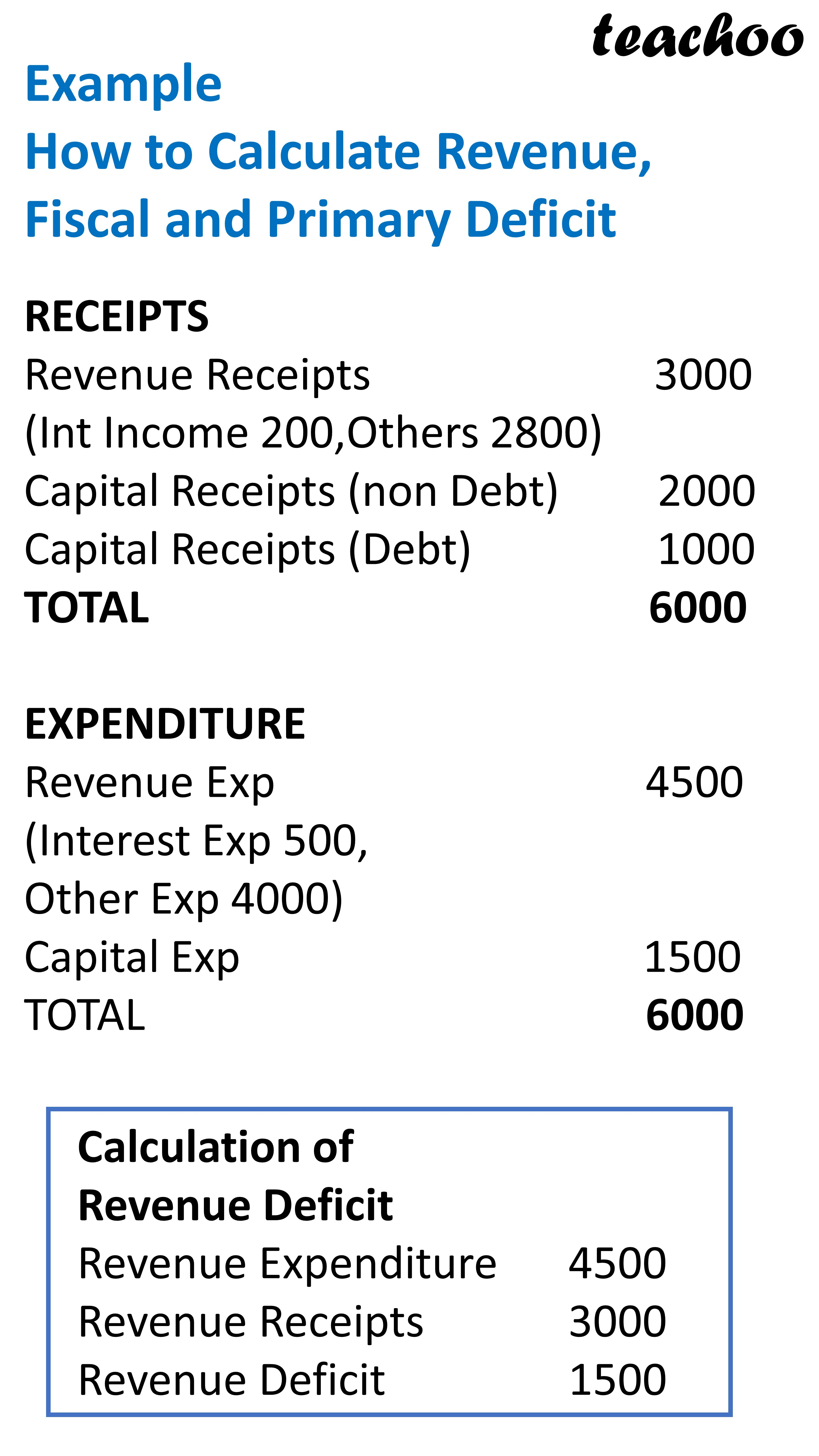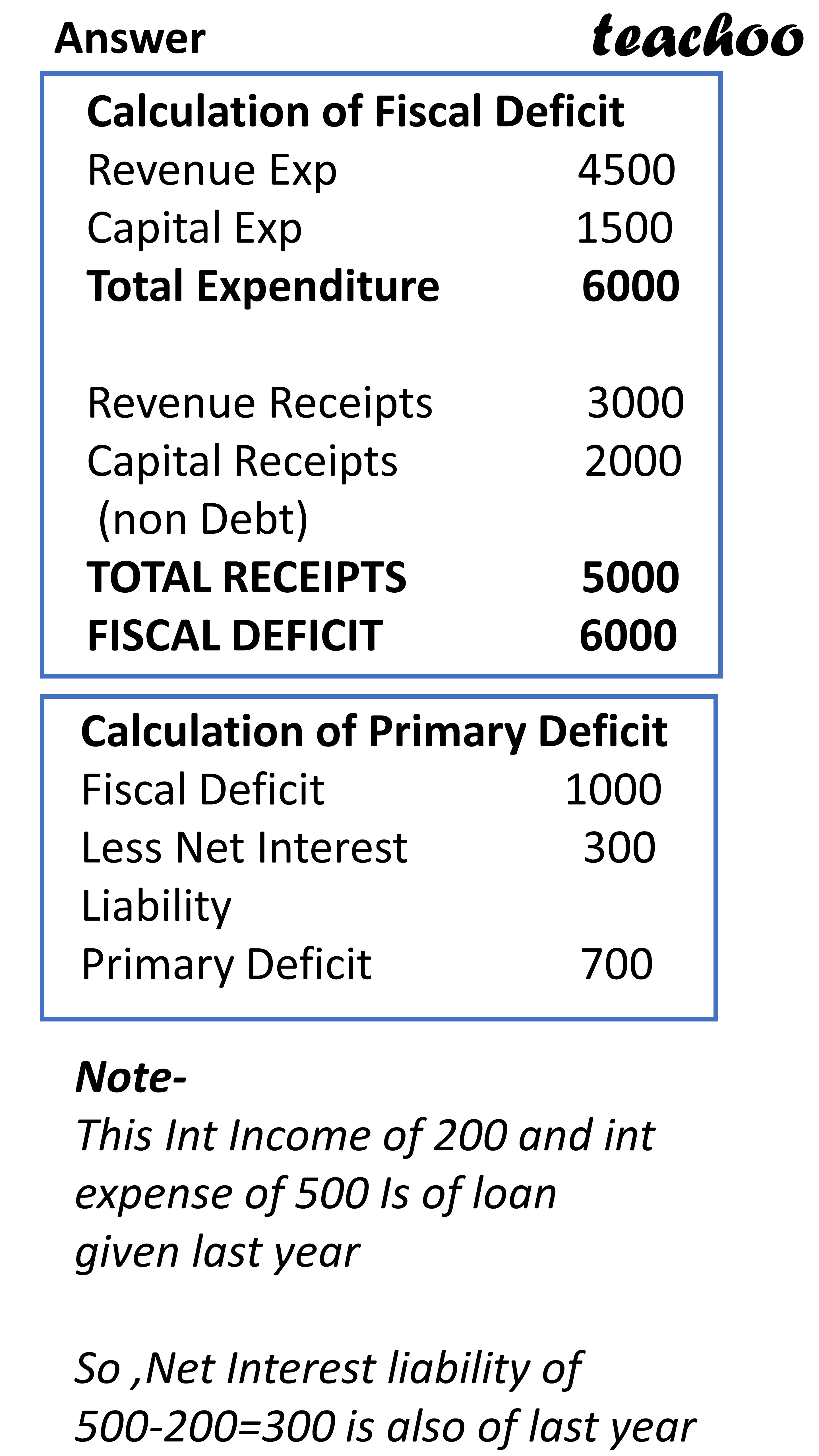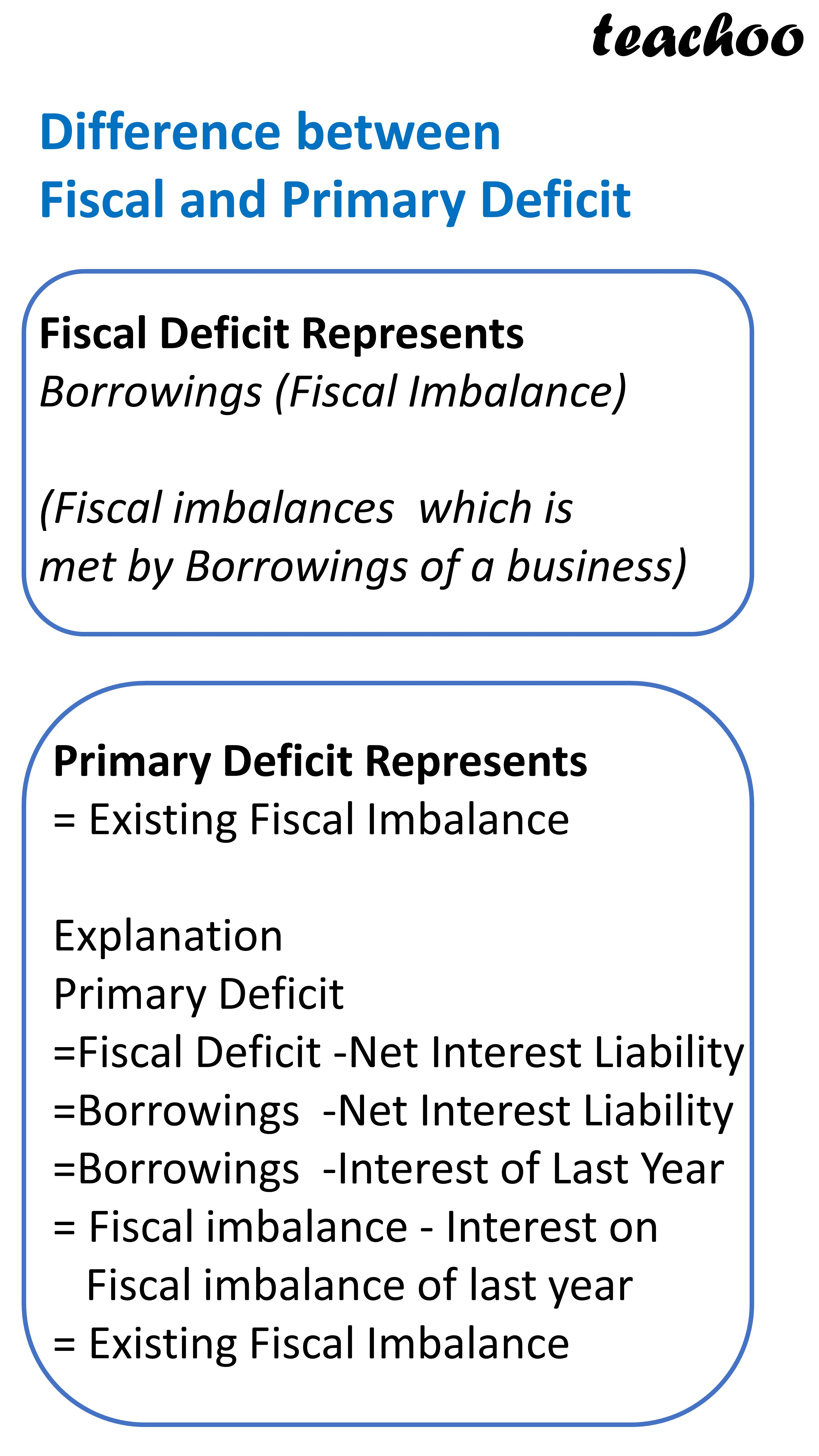Chapter 5 - Government Budget

Economics Class 12
Macroeconomics

## What is Primary Deficit?

It is difference between

Fiscal Deficit of Current year

and

Net Interest Payments

#### FORMULA

PRIMARY DEFICIT

= FISCAL DEFICIT - NET INTEREST LIABILITY

=FISCAL DEFICIT - (INT PAYMENT ON LOAN TAKEN - INT RECEIVED ON LOAN GIVEN)### NCERT Questions

No questions in this part

### Other Books

#### Question 1

In the following questions, select the correct answers:

Primary defect in a government budget is:

1. Revenue expenditure - Revenue receipts
2. Total expenditure - Total receipts
3. Revenue defect - Interest Payments
4. Fiscal deficit - Interest Payments

#### Question 2

Which of the following statements is true?

1. Fiscal deficit is the difference between total expenditure and total receipts
2. Primary Deficit is the difference between total receipt and interest payments
3. Fiscal Deficit is the sum of Primary Deficit and Interest Payments

#### Question 3

Primary deficit in a government budget is zero, when _____.

1. Revenue Deficit is zero
2. Net Interest Payments are zero
3. Fiscal defict is zero
4. Fiscal defict is equal to interest payment

Learn in your speed, with individual attention - Teachoo Maths 1-on-1 Class

### Transcript

Different Types of Deficit Revenue Deficit Fiscal Deficit Primary Deficit Formula Revenue Exp less Revenue Receipts Formula Total Exp less Total Receipt (non debt) Formula Fiscal Deficit less Net Interest Liability These 2 we have already studied This we will study now Example How to Calculate Revenue, Fiscal and Primary Deficit RECEIPTS Revenue Receipts 3000 (Int Income 200,Others 2800) Capital Receipts (non Debt) 2000 Capital Receipts (Debt) 1000 TOTAL 6000 EXPENDITURE Revenue Exp 4500 (Interest Exp 500, Other Exp 4000) Capital Exp 1500 TOTAL 6000 Calculation of Revenue Deficit Revenue Expenditure 4500 Revenue Receipts 3000 Revenue Deficit 1500 Answer Calculation of Fiscal Deficit Revenue Exp 4500 Capital Exp 1500 Total Expenditure 6000 Revenue Receipts 3000 Capital Receipts 2000 (non Debt) TOTAL RECEIPTS 5000 FISCAL DEFICIT 6000 Calculation of Primary Deficit Fiscal Deficit 1000 Less Net Interest 300 Liability Primary Deficit 700 Note- This Int Income of 200 and int expense of 500 Is of loan given last year So ,Net Interest liability of 500-200=300 is also of last year Difference between Fiscal and Primary Deficit Fiscal Deficit Represents Borrowings (Fiscal Imbalance) (Fiscal imbalances which is met by Borrowings of a business) Primary Deficit Represents = Existing Fiscal Imbalance Explanation Primary Deficit =Fiscal Deficit -Net Interest Liability =Borrowings -Net Interest Liability =Borrowings -Interest of Last Year = Fiscal imbalance - Interest on Fiscal imbalance of last year = Existing Fiscal Imbalance